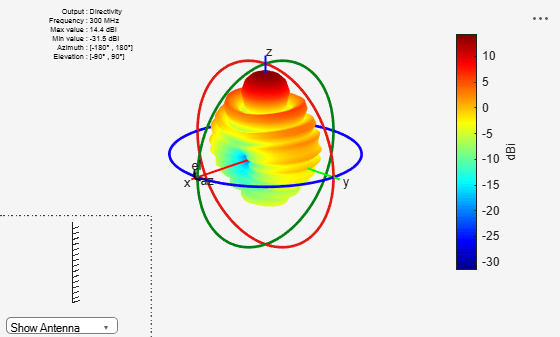yagiUda

Create Yagi-Uda array antenna

Description

The yagiUda class creates a classic Yagi-Uda array comprised of an exciter, reflector, and N- directors along the z-axis. The reflector and directors create a traveling wave structure that results in a directional radiation pattern.

The exciter, reflector, and directors have equal widths and are related to the diameter of an equivalent cylindrical structure by the equation

$w=2d=4r$

where:

• d is the diameter of equivalent cylinder

• r is the radius of equivalent cylinder

For a given cylinder radius, use the cylinder2strip utility function to calculate the equivalent width. A typical Yagi-Uda antenna array uses folded dipole as an exciter, due to its high impedance. The Yagi-Uda is center-fed and the feed point coincides with the origin. In place of a folded dipole, you can also use a planar dipole as an exciter.Creation

Description

yu = yagiUda creates a half-wavelength Yagi-Uda array antenna along the Z-axis. The default Yagi-Uda uses folded dipole as three directors, one reflector, and a folded dipole as an exciter. By default, the dimensions are chosen for an operating frequency of 300 MHz.

example

yu = yagiUda(Name,Value) creates a half-wavelength Yagi-Uda array antenna, with additional properties specified by one or more name-value pair arguments. Name is the property name and Value is the corresponding value. You can specify several name-value pair arguments in any order as Name1, Value1, ..., NameN, ValueN. Properties not specified retain default values.

Properties

expand all

Antenna Type used as exciter, specified as the comma-separated pair consisting of 'Exciter' and an object.

Example: 'Exciter',dipole

Total number of director elements, specified as a scalar.

Note

Number of director elements should be less than or equal to 20.

Example: 'NumDirectors',13

Data Types: double

Director length, specified as a scalar or vector in meters.

Example: 'DirectorLength',[0.4 0.5]

Data Types: double

Spacing between directors, specified as a scalar or vector in meters.

Example: 'DirectorSpacing',[0.4 0.5]

Data Types: double

Reflector length, specified as a scalar in meters.

Example: 'ReflectorLength',0.3

Data Types: double

Spacing between exciter and reflector, specified as a scalar in meters.

Example: 'ReflectorSpacing', 0.4

Data Types: double

Type of the metal used as a conductor, specified as a metal material object. You can choose any metal from the MetalCatalog or specify a metal of your choice. For more information, see metal. For more information on metal conductor meshing, see Meshing.

Example: m = metal('Copper'); 'Conductor',m

Example: m = metal('Copper'); ant.Conductor = m

Lumped elements added to the antenna feed, specified as a lumped element object. For more information, see lumpedElement.

Example: 'Load',lumpedelement. lumpedelement is the object for the load created using lumpedElement.

Tilt angle of the antenna, specified as a scalar or vector with each element unit in degrees. For more information, see Rotate Antennas and Arrays.

Example: 'Tilt',90

Example: ant.Tilt = 90

Example: 'Tilt',[90 90],'TiltAxis',[0 1 0;0 1 1] tilts the antenna at 90 degrees about the two axes defined by the vectors.

Note

The wireStack antenna object only accepts the dot method to change its properties.

Data Types: double

Tilt axis of the antenna, specified as:

• Three-element vector of Cartesian coordinates in meters. In this case, each coordinate in the vector starts at the origin and lies along the specified points on the X-, Y-, and Z-axes.

• Two points in space, each specified as three-element vectors of Cartesian coordinates. In this case, the antenna rotates around the line joining the two points in space.

• A string input describing simple rotations around one of the principal axes, 'X', 'Y', or 'Z'.

Example: 'TiltAxis',[0 1 0]

Example: 'TiltAxis',[0 0 0;0 1 0]

Example: ant.TiltAxis = 'Z'

Note

The wireStack antenna object only accepts the dot method to change its properties.

Data Types: double

Object Functions

 show Display antenna or array structure; display shape as filled patch info Display information about antenna or array axialRatio Axial ratio of antenna beamwidth Beamwidth of antenna charge Charge distribution on metal or dielectric antenna or array surface current Current distribution on metal or dielectric antenna or array surface design Design prototype antenna or arrays for resonance around specified frequency efficiency Radiation efficiency of antenna EHfields Electric and magnetic fields of antennas; Embedded electric and magnetic fields of antenna element in arrays impedance Input impedance of antenna; scan impedance of array mesh Mesh properties of metal or dielectric antenna or array structure meshconfig Change mesh mode of antenna structure optimize Optimize antenna or array using SADEA optimizer pattern Radiation pattern and phase of antenna or array; Embedded pattern of antenna element in array patternAzimuth Azimuth pattern of antenna or array patternElevation Elevation pattern of antenna or array rcs Calculate and plot radar cross section (RCS) of platform, antenna, or array returnLoss Return loss of antenna; scan return loss of array sparameters Calculate S-parameter for antenna and antenna array objects vswr Voltage standing wave ratio of antenna

Examples

collapse all

Create and view a Yagi-Uda array antenna with 13 directors.

y = yagiUda('NumDirectors',13);
show(y)Plot the radiation pattern of a Yagi-Uda array antenna at a frequency of 300 MHz.

y = yagiUda('NumDirectors',13);
pattern(y,300e6)Calculate the width of the strip approximation to a cylinder of radius 20 mm.

w = cylinder2strip(20e-3)
w = 0.0800

 Balanis, C.A. Antenna Theory. Analysis and Design, 3rd Ed. New York: Wiley, 2005.